Fractions on a Number Line 2
Set 2 (20 Questions)
Identify and represent on a number line decimals, fractions, mixed numbers, and positive and negative integers.
From Mr. Anker Tests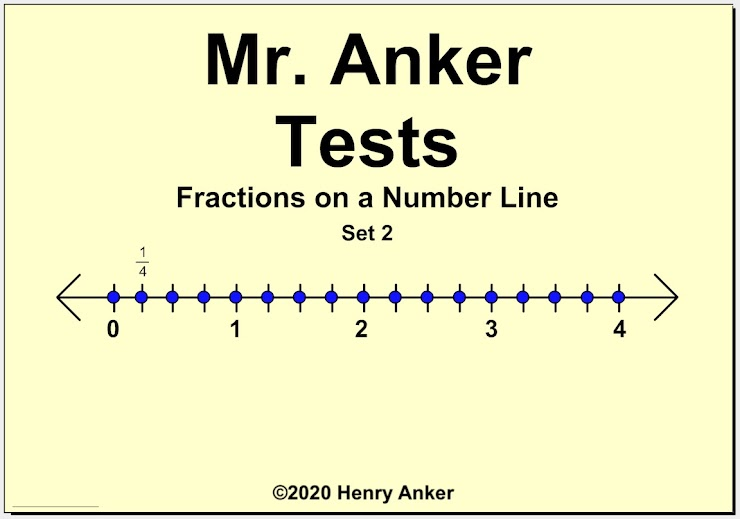Fractions on a Number Line Help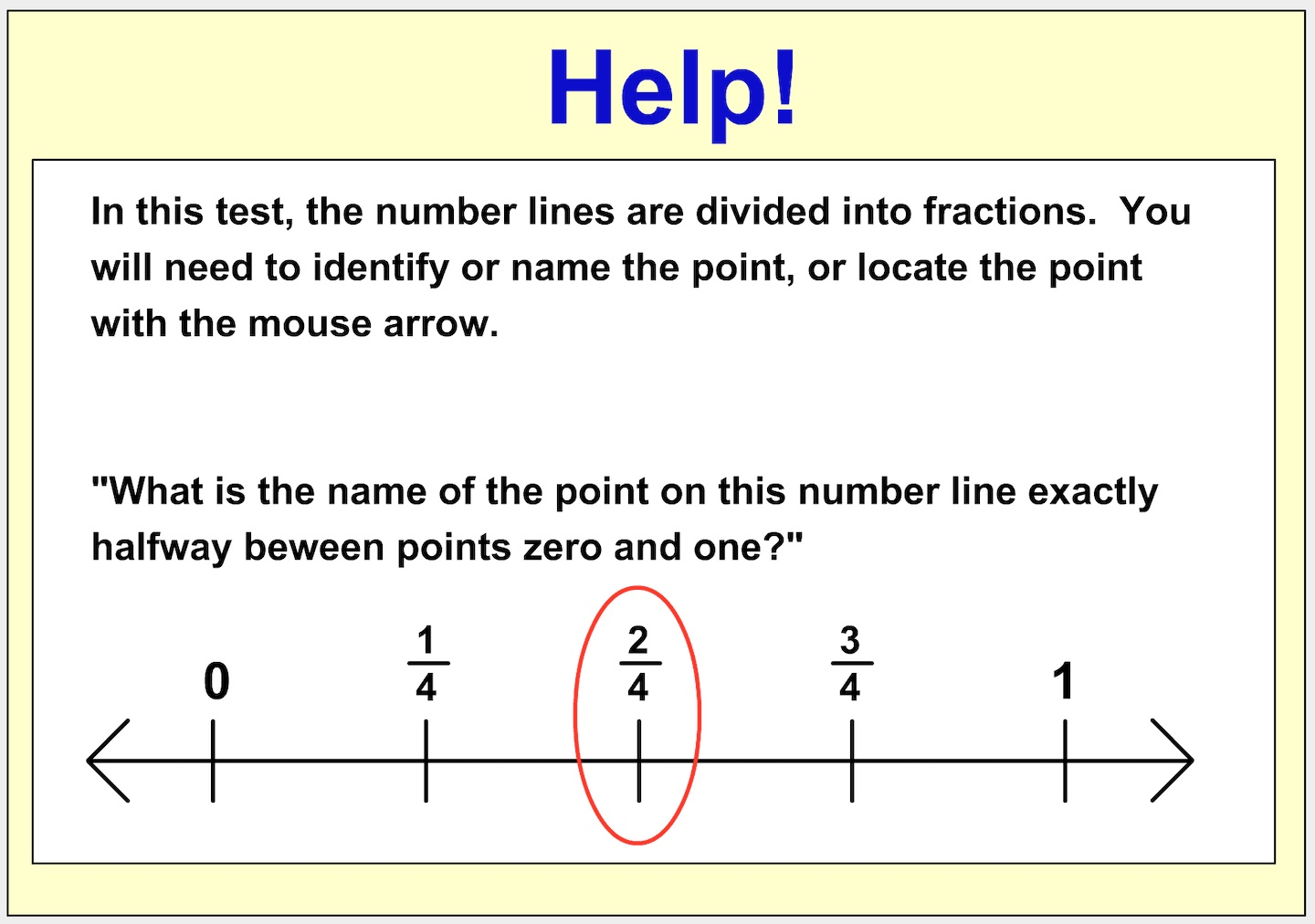1.  The same point on a number line can sometimes be named by equivalent fractions. Which fraction from the dropdown menu is equivalent to 2/3? *
1 point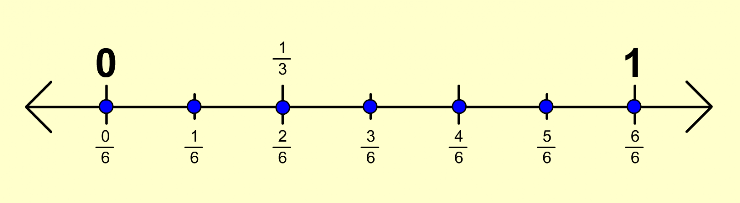2. The same point on a number line can sometimes be named by equivalent fractions. Which fraction from the dropdown menu is equivalent to 1/2? *
1 point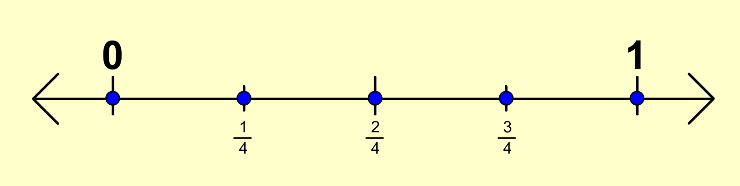3. Point D is in which position on the number line? *
1 point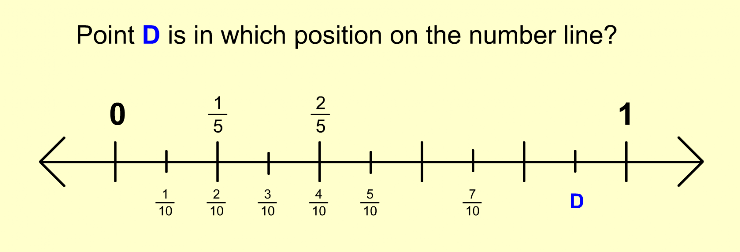4.  Which of the statements below is false? *
1 point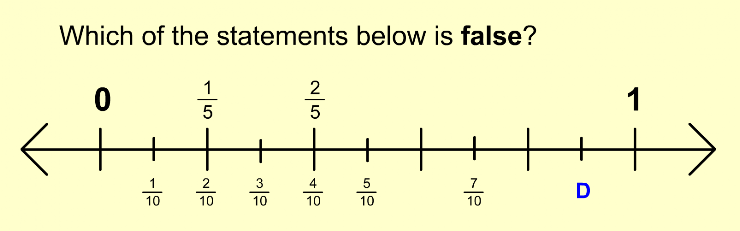5.  Which of the choices below would not be a correct name for Point S on the number line? *
1 point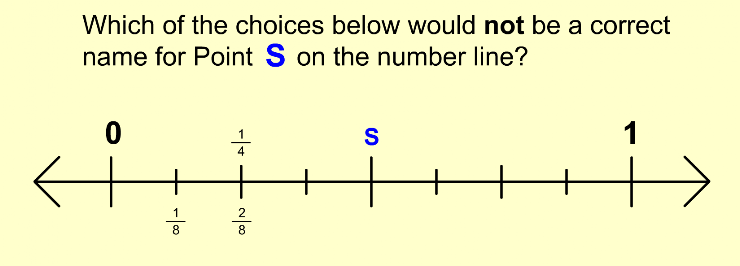6. How is this number line divided? *
1 point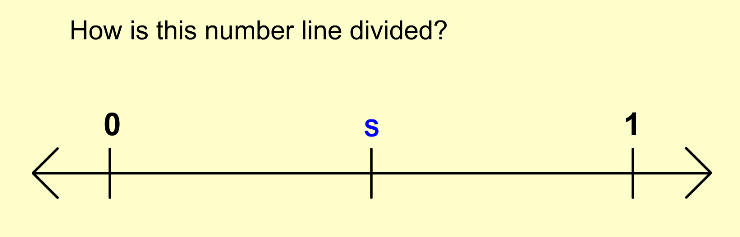7. Which of the statements below is false? *
1 point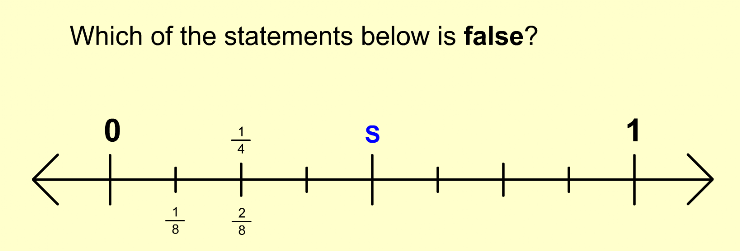8. Which mixed number would name the blue point? *
1 point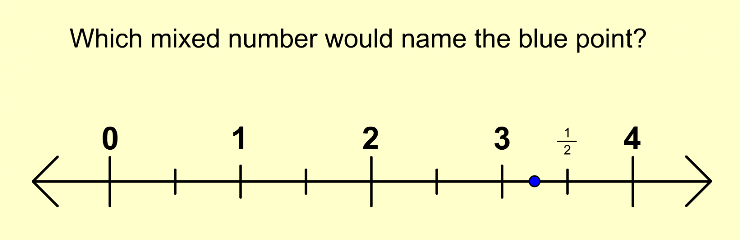9. Which letter represents 3 & 1/4 on the number line? *
1 point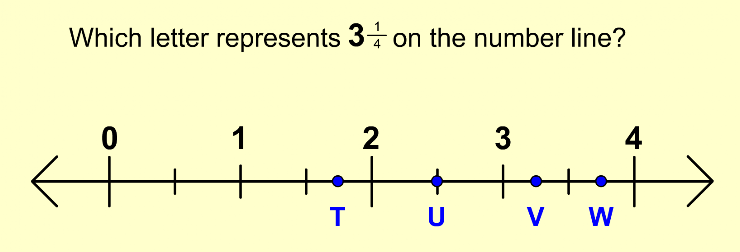10.  Which letter represents 3 & 1/2 on the number line? *
1 point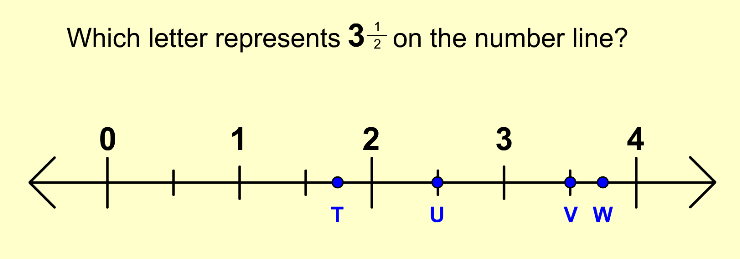11. Which letter represents 3 & 3/4 on the number line? *
1 point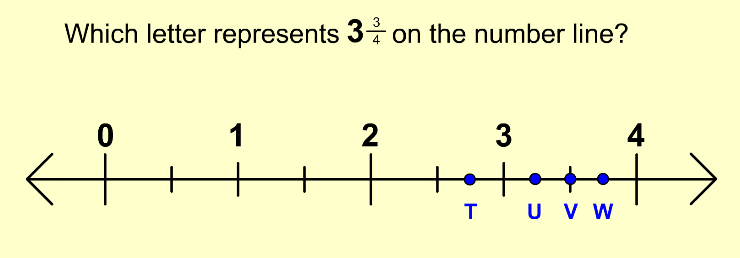12. Which lettered point represents a value less than 3 on the number line? *
1 point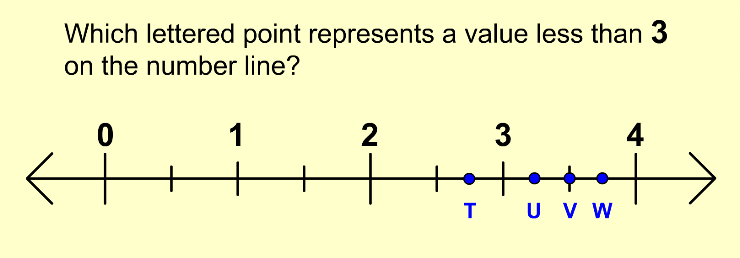13. What improper fraction is equivalent to the whole number 2? *
1 point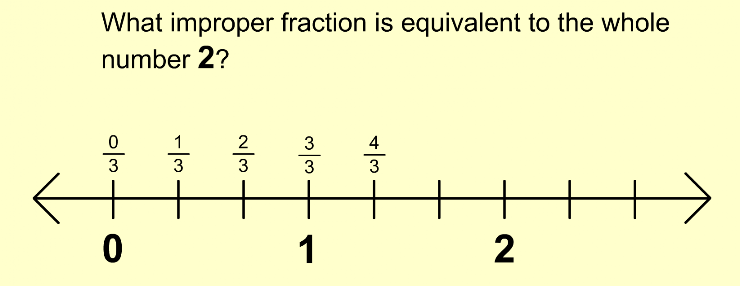14.  On which lettered point does 7/3 occur on this number line? *
1 point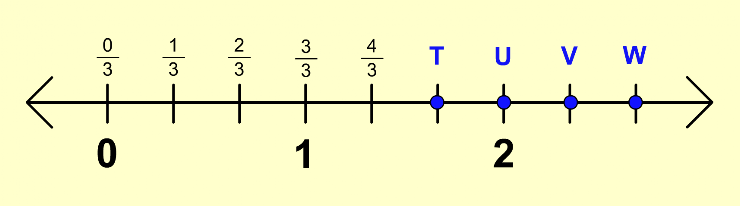15.  On which lettered point does 5/2 occur on this number line? *
1 point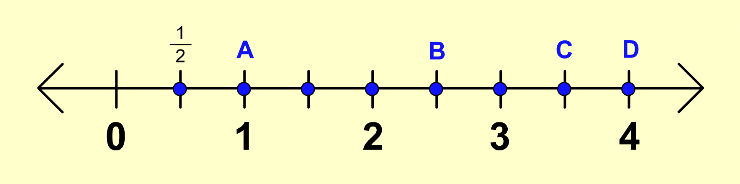16.  Use the dropdown menu to indicate which lettered point occurs at 5/4 on this number line. *
1 point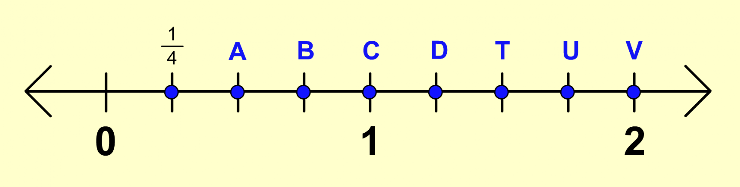17. Use the dropdown menu to indicate which lettered point occurs at 1 & 3/7 on this number line. *
1 point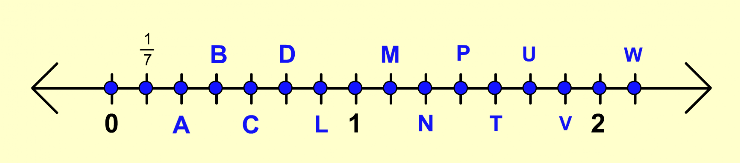18.  Where does the point 12/5 occur on this number line? *
1 point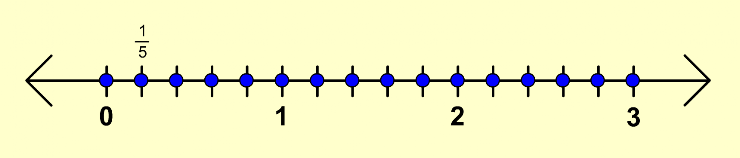19. Where does the point 3 & 3/4 occur on this number line? *
1 point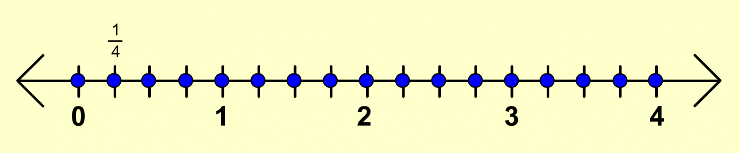20. What whole number is equal in value to the improper fraction 12/4? *
1 point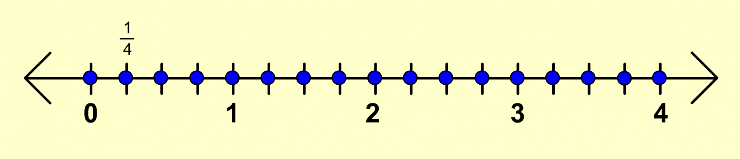Submit
Clear form